# GSEB Solutions Class 10 Maths Chapter 11 Constructions Ex 11.1

Gujarat Board GSEB Solutions Class 10 Maths Chapter 11 Constructions Ex 11.1 Textbook Questions and Answers.

## Gujarat Board Textbook Solutions Class 10 Maths Chapter 11 Constructions Ex 11.1

Question 1.
Draw a line segment of length 7.6 cm and divide it in the ratio 5 : 8. Measure the two parts.
Solution:
Steps of Construction
1. Draw AB = 7.6 cm
2. At A, draw an acute angle ∠BAX below BA.
3. On AX mark 13 (5 + 8) arcs A1, A2, …, A13.
4. Join B to A13.
5. From A5, draw A5C || A13B.
AC : CO = 5 : 8.Justification:
In ΔABA13, A5C || A13
⇒ $$\frac {AC}{BC}$$ = $$\frac{\mathrm{AA}_{5}}{\mathrm{A}_{5} \mathrm{A}_{13}}$$ [Thales Theorem]
But $$\frac{\mathrm{AA}_{5}}{\mathrm{A}_{5} \mathrm{A}_{13}}$$ = $$\frac {5}{8}$$
[By construction]
∴ $$\frac {AC}{BC}$$ = $$\frac {5}{8}$$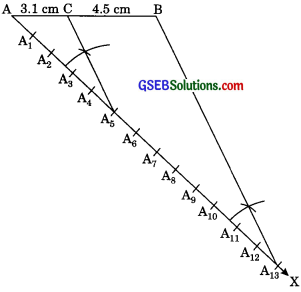Question 2.
Construct a triangle of sides 4 cm, 5 cm and 6 cm and then a triangle similar to it whose sides are of the corresponding sides of the first triangle.
Solution:
Steps of Construction:
1. Draw ΔABC with AC = 6cm, AB = 5 cm, BC = 4 cm.
2. At A1 draw an acute angle∠CAX below the base AC.
3. Mark 3 arcs on AX such that AA1 = A1A2 = A2A3.
4. Join A3C.
5. Draw A2C’ || A3C
6. From C’ draw B’C’ || BC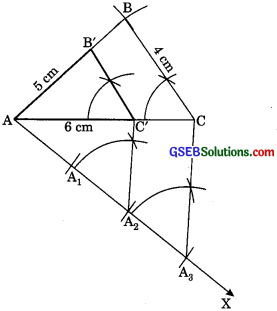Thus, ΔAB’C’ is the required triangle.

Justification:
ΔAB’C’ ∼ ΔABC [AA similarity Rule]
$$\frac{\mathrm{AB}^{\prime}}{\mathrm{AB}}=\frac{\mathrm{AC}^{\prime}}{\mathrm{AC}}=\frac{\mathrm{B}^{\prime} \mathrm{C}^{\prime}}{\mathrm{BC}}$$ ……….(1)
[Sides are proportional]
But $$\frac{A C^{\prime}}{A C}=\frac{A A_{2}}{A A_{3}}=\frac{2}{3}$$ ………..(2)
From (1) and (2)
But $$\frac{A B^{\prime}}{A B}=\frac{A C^{\prime}}{A C}=\frac{B^{\prime} C^{\prime}}{B C}=\frac{2}{3}$$Question 3.
Construct a triangle with sides 5 cm, 6 cm and 7 cm and then another triangle whose sides are $$\frac {5}{8}$$ of the corresponding sides of the first triangle.
Solution:
1. Construct a ΔABC with AB = 7 cm, AC = 5 cm, BC = 6 cm
2. At A draw an acute ∠BAX below AB.
3. On AX, mark 7 arcs A1, A2, …, A7 such that
AA1 = A1A2 =…=A6A7.
4. Join A5B.
5. From A7 draw A7B’ || A5B meeting AB at B’ (extent AB to B’)
6. From B’ draw B’C’ || BC meeting AC at C’ [extend AC to C’]
AB’C’ is the required triangle each of whose sides is $$\frac {5}{8}$$ of the corresponding sides of ΔABC.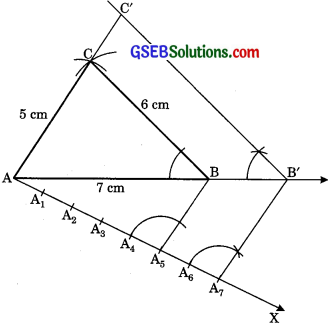Justification:
$$\frac{C^{\prime} A}{C A}=\frac{A B^{\prime}}{A B}=\frac{C^{\prime} B^{\prime}}{C B}$$ ………..(1)
[Sides are proportional]
But $$\frac{\mathrm{AB}^{\prime}}{\mathrm{AB}}=\frac{\mathrm{AA}_{7}}{\mathrm{AA}_{5}}$$ = $$\frac {7}{5}$$ ………(2)
From (1) and (2)
$$\frac{C^{\prime} A}{C A}=\frac{A B^{\prime}}{A B}=\frac{C^{\prime} B^{\prime}}{C B}$$ = $$\frac {7}{5}$$

Question 4.
Construct an isosceles triangle whose base is 8 cm and altitude is 4 cm and then another triangle whose sides are 1 times the corresponding sides of the isosceles triangle. (CBSE 2017)
Solution:
Steps of Construction:
1. Draw a line segment BC = 8 cm.
2. Draw its perpendicular bisector AD (4 cm).
3. Joining AB and AC, we get isosceles triangle ABC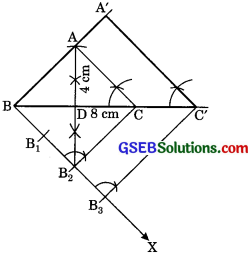4. Construct an acute ∠CBX.
5. Along BX cut 3 arcs B1, B2 and B3 such that
BB1 = B1B2 = B2B3.
6. Join C to B2 and draw a line B3C’ || B2C [extend BC to C’]
7. From C’ draw C’A’ || CA [extend BA to A’]
∴ ΔA’BC’ is a required triangle.

Justification:
Now C’A’ || CA (By construction)
∴ ΔABC ∼ ΔA’BC’ [AA similarity criteria]
∴ $$\frac{\mathrm{A}^{\prime} \mathrm{B}}{\mathrm{AB}}=\frac{\mathrm{A}^{\prime} \mathrm{C}^{\prime}}{\mathrm{AC}}=\frac{\mathrm{BC}^{\prime}}{\mathrm{BC}}$$ [Sides are proportional]
Now B3C’ || B2C (By construction)
∴ ΔBB3C’ – ΔBB2C [AA similarity criteria]
∴ $$\frac{\mathrm{BC}^{\prime}}{\mathrm{BC}}=\frac{\mathrm{BB}_{3}}{\mathrm{BB}_{2}}$$ [Thales theorem]
But $$\frac{\mathrm{BB}_{3}}{\mathrm{BB}_{2}}=\frac{3}{2}$$ (By construction]
∴ $$\frac{B C^{\prime}}{B C}=\frac{3}{2}$$
∴ $$\frac{\mathrm{A}^{\prime} \mathrm{B}}{\mathrm{AB}}=\frac{\mathrm{A}^{\prime} \mathrm{C}^{\prime}}{\mathrm{AC}}=\frac{\mathrm{BC}^{\prime}}{\mathrm{BC}}=\frac{3}{2}$$Question 5.
Draw a triangle ABC with side BC = 6 cm, AB = 5 cm and ∠ABC = 60°. Then construct a triangle whose sides are $$\frac{3}{4}$$ of the corresponding sides of the ABC. (CBSE 2015)
Solution:
Steps of Construction:
1. Draw a line segment BC = 6 cm.
2. At B draw ∠CBY = 60° on which take AB = 5 cm.
3. Join AC. ΔABC is required triangle.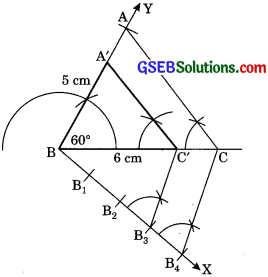4. From B, draw an acute ∠CBX downwards.
5. Mark four arcs B1, B2, B3, B4 on BX such that BB1 = B1B2 = B2B3 = B3B4.
6. Join B4C and from B3 draw B3C’ B4C.
7. From C’, draw A’C’ || AC.
When ΔA’BC’ is the required triangle whose sides are $$\frac{3}{4}$$ of the corresponding sides of ΔABC.

Justification:
A’C’ || AC (By construction)
∴ ΔA’BC’ ∼ ΔABC (AA similarity Rule)
∴ $$\frac{\mathrm{BC}^{\prime}}{\mathrm{BC}}=\frac{\mathrm{BB}_{3}}{\mathrm{BB}_{2}}$$ ………..(1)
[Sides are proportional]
But $$\frac{\mathrm{BC}^{\prime}}{\mathrm{BC}}=\frac{\mathrm{BB}_{3}}{\mathrm{BB}_{4}}=\frac{3}{4}$$ ………….(2)
From (1) and (2)
$$\frac{\mathrm{A}^{\prime} \mathrm{B}}{\mathrm{AB}}=\frac{\mathrm{BC}^{\prime}}{\mathrm{BC}}=\frac{\mathrm{A}^{\prime} \mathrm{C}^{\prime}}{\mathrm{AC}}=\frac{3}{4}$$Question 6.
Draw a triangle ABC with side BC = 7 cm, ∠B = 45°, ∠A = 105°. Then construct a triangle whose sides are $$\frac{3}{4}$$ times the corresponding sides of the ΔABC. (CBSE 2012, 2017)
Solution:
Steps of Construction:
1. Draw a ABC with side BC = 7 cm, ∠B = 45°, ∠C = 30°
[∠C = 180° – 45° – 105° = 180° – 150° = 30°]
2. At B, draw an acute ∠CBX below BC.
3. On BX, mark the arcs B1, B2, B3, B4 such
that BB1 = B1B2 = B2B3 = B1B4.
4. Join B3C.
5. Draw B4C’|| B3C [Extend BC to C’]
6. Draw C’A’ || CA [Extend BA to A’]
∴ ΔA’BC’ is the required triangle.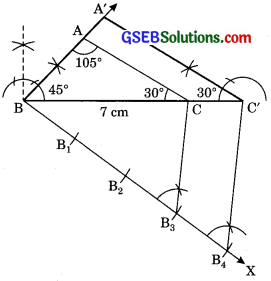Justification:
C’A’ || CA (By construction)
∴ ΔABC ∼ ΔA’BC’ (AA similarity Criteria)
$$\frac{\mathrm{A}^{\prime} \mathrm{B}}{\mathrm{AB}}=\frac{\mathrm{A}^{\prime} \mathrm{C}^{\prime}}{\mathrm{AC}}=\frac{\mathrm{BC}^{\prime}}{\mathrm{BC}}$$ ………(1) [Corresponding sides are proportional]
Now B4C’ || B3C (By construction)
∴ $$\frac{\mathrm{BC}^{\prime}}{\mathrm{BC}}=\frac{\mathrm{BB}_{4}}{\mathrm{BB}_{3}}$$ ……..(2)
[Thales theorem]
But = $$\frac{B B_{4}}{B B_{3}}=\frac{4}{3}$$ ………(3) (By construction)
From (1) (2) and (3)
$$\frac{\mathrm{A}^{\prime} \mathrm{B}}{\mathrm{AB}}=\frac{\mathrm{A}^{\prime} \mathrm{C}^{\prime}}{\mathrm{AC}}=\frac{\mathrm{BC}^{\prime}}{\mathrm{BC}}=\frac{4}{3}$$Question 7.
Draw a right triangle in which the sides (other than hypotenuse) are of lengths 4 cm and 3 cm. Then construct another triangle whose sides are times the corresponding sides of the given triangle. (CBSE 2010)
Solution:
1. Draw a line segment AB = 4 cm.
2. By making right angle at B. Draw BC = 3 cm.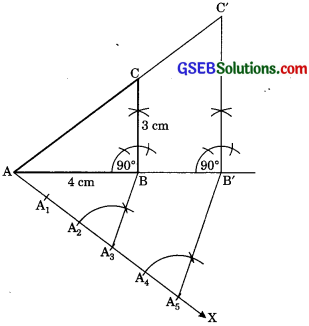3. Join AC. ΔABC is the required right-angled triangle.
4. At A, Draw acute angle ∠BAX downwards.
5. On AX, mark 5 arcs A1, A2, A3, A4, A5 such
that AA1 = A1A2 = … = A4A5
6. Join A3B.
7. Draw A5B’|| A3B [Extend AB to B’]
8. Draw B1C’ || BC [Extend AC to C’]
Hence ΔAB’C’ is the required triangle

Justification:
ΔABC ∼ ΔABC(AA similarity Rule)
$$\frac{A B^{\prime}}{A B}=\frac{A C^{\prime}}{A C}=\frac{B^{\prime} C^{\prime}}{B C}$$ ……..(1) [Sides are proportional]
But $$\frac{A B^{\prime}}{A B}=\frac{A A_{5}}{A A_{3}}=\frac{5}{3}$$ ……(2)
From (1) and (2)
$$\frac{A B^{\prime}}{A B}=\frac{A C^{\prime}}{A C}=\frac{B^{\prime} C^{\prime}}{B C}=\frac{5}{3}$$